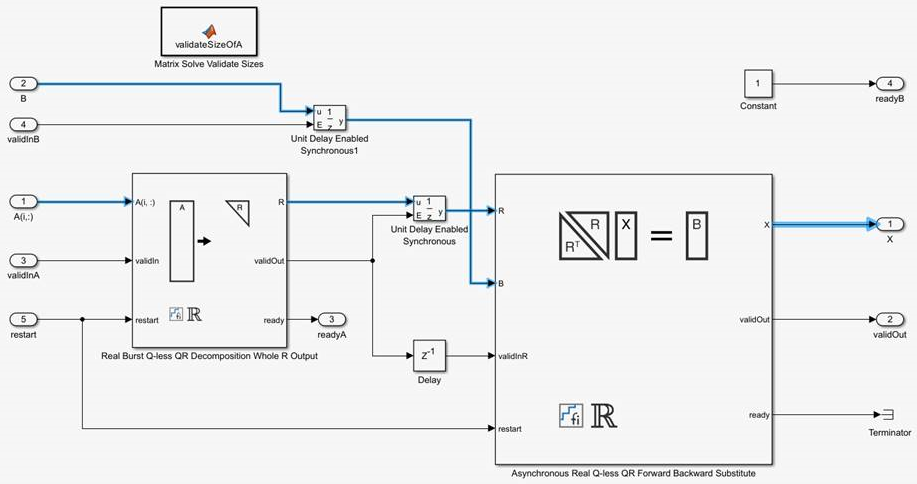## Choose a Block for HDL-Optimized Fixed-Point Matrix Operations

You can use the Fixed-Point Designer™ HDL Support library of blocks to perform fixed-point matrix operations and generate efficient HDL code. These blocks model design patterns for systems of linear equations and core matrix operations, such as QR decomposition, for hardware-efficient implementation on FPGAs.

This topic discusses how to choose an appropriate block from the Fixed-Point Designer HDL Support library for your application.

### Define the Problem to Solve

First, define the math problem that you need to solve and the algorithm to use.

#### Linear System Solvers

Use the Linear System Solver library of blocks to solve these systems of linear equations.

OperationBlocksDescription
Ax = BMatrix Solve Using QR Decomposition blocks

Use QR decomposition to solve the system of linear equations Ax = B. To compute x = A-1, set B to be the identity matrix.

A'AX = BMatrix Solve Using Q-less QR Decomposition blocks

Solve the system of linear equations A'AX = B using QR decomposition, without computing Q.

A'AX = BMatrix Solve Using Q-less QR Decomposition with Forgetting Factor blocks

Solve the system of linear equations A'AX = B using QR decomposition, without computing Q. A is an infinitely tall matrix representing streaming data.

#### Matrix Factorizations

Use the Matrix Factorizations library of blocks to perform QR decomposition, also known as QR factorization.

OperationBlocksDescription

QR decomposition

QR Decomposition blocks

Use QR decomposition to compute R and C=Q'B, where QR=A, where A and B are your input matrices. The least-squares solution to Ax=B is x=R\C. R is an upper-triangular matrix and Q is an orthogonal matrix. To compute C=Q', set B to be the identity matrix.

QR decomposition without computing Q

Q-less QR Decomposition blocks

Use Q-less QR decomposition to compute the economy size upper-triangular R factor of the QR decomposition A = QR, without computing Q. The solution to A'Ax = B is x = R\R'\b.

QR decomposition without computing Q and an infinite number of rows

Q-less QR Decomposition with Forgetting Factor blocks

Use Q-less QR decomposition to compute the economy size upper-triangular R factor of the QR decomposition A = QR, without computing Q. A is an infinitely tall matrix representing streaming data.

### Choose an Architecture

Blocks in the Fixed-Point Designer HDL Support > Matrices and Linear Algebra library are available in burst and partial-systolic implementations. Partial-systolic implementations prioritize speed of computations over space constraints, while burst implementations prioritize space constraints at the expense of speed of the operations. The following table illustrates the tradeoffs between the implementations available for matrix decompositions and solving systems of linear equations.

Partial-SystolicCO(m)O(n2)
BurstO(n)O(mn2)O(n)

Where C is a constant proportional to the word length of the data, m is the number of rows in matrix A, and n is the number of columns in matrix A.

### Linear System Solvers: Select Synchronous or Asynchronous Operation

The Matrix Solve Using QR Decomposition blocks operate synchronously. These blocks first decompose the input A and B matrices into R and C matrices using a QR decomposition block. Then, a back substitute block computes RX = C. The input A and B matrices propagate through the system in parallel, in a synchronized way.The Matrix Solve Using Q-less QR Decomposition blocks operate asynchronously. First, Q-less QR decomposition is performed on the input A matrix and the resulting R matrix is put into a buffer. Then, a forward backward substitution block uses the input B matrix and the buffered R matrix to compute R'RX = B. Because the R and B matrices are stored separately in buffers, the upstream Q-less QR decomposition block and the downstream Forward Backward Substitute block can run independently. The Forward Backward Substitute block starts processing when the first R and B matrices are available. Then it runs continuously using the latest buffered R and B matrices, regardless of the status of the Q-less QR Decomposition block. For example, if the upstream block stops providing A and B matrices, the Forward Backward Substitute block continues to generate the same output using the last pair of R and B matrices.The Burst (Asynchronous) Matrix Solve Using Q-less QR Decomposition blocks are available in both synchronous and asynchronous operation variants, as denoted by the block name.

### Data Complexity

All blocks in the Fixed-Point Designer HDL Support > Matrices and Linear Algebra library are available in real and complex variants. Choose the real or complex variant of the block based on the complexity of your data.

### Hardware Control Signals

#### Restart Signal

Some blocks in the Fixed-Point Designer HDL Support > Matrices and Linear Algebra library provide an input reset signal that clears internal states.

#### AMBA AXI Handshake Process

Blocks in the Fixed-Point Designer HDL Support > Matrices and Linear Algebra library use the AMBA AXI handshake protocol . The `valid/ready` handshake process is used to transfer data and control information. This two-way control mechanism allows both the manager and subordinate to control the rate at which information moves between manager and subordinate. A `valid` signal indicates when data is available. The `ready` signal indicates that the block can accept the data. Transfer of data occurs only when both the `valid` and `ready` signals are high.